Minima/ maxima (statement questions) - Geometry questions

Chapter 6 Class 12 Application of Derivatives
Concept wise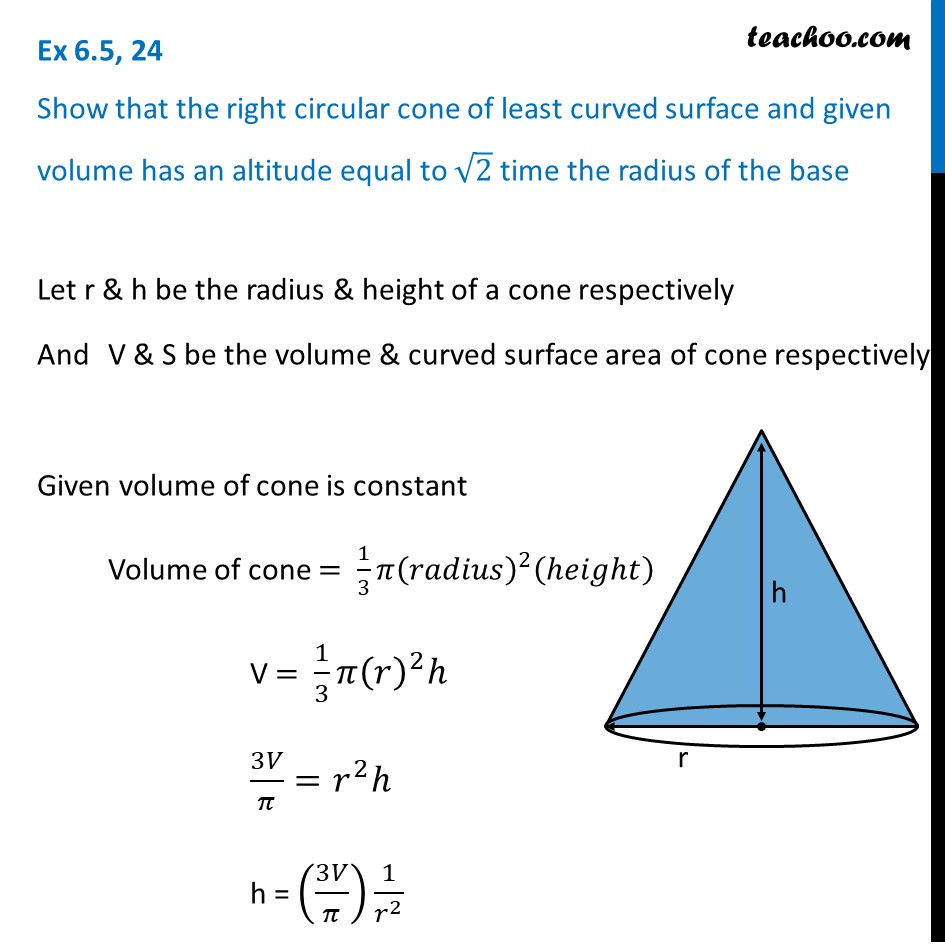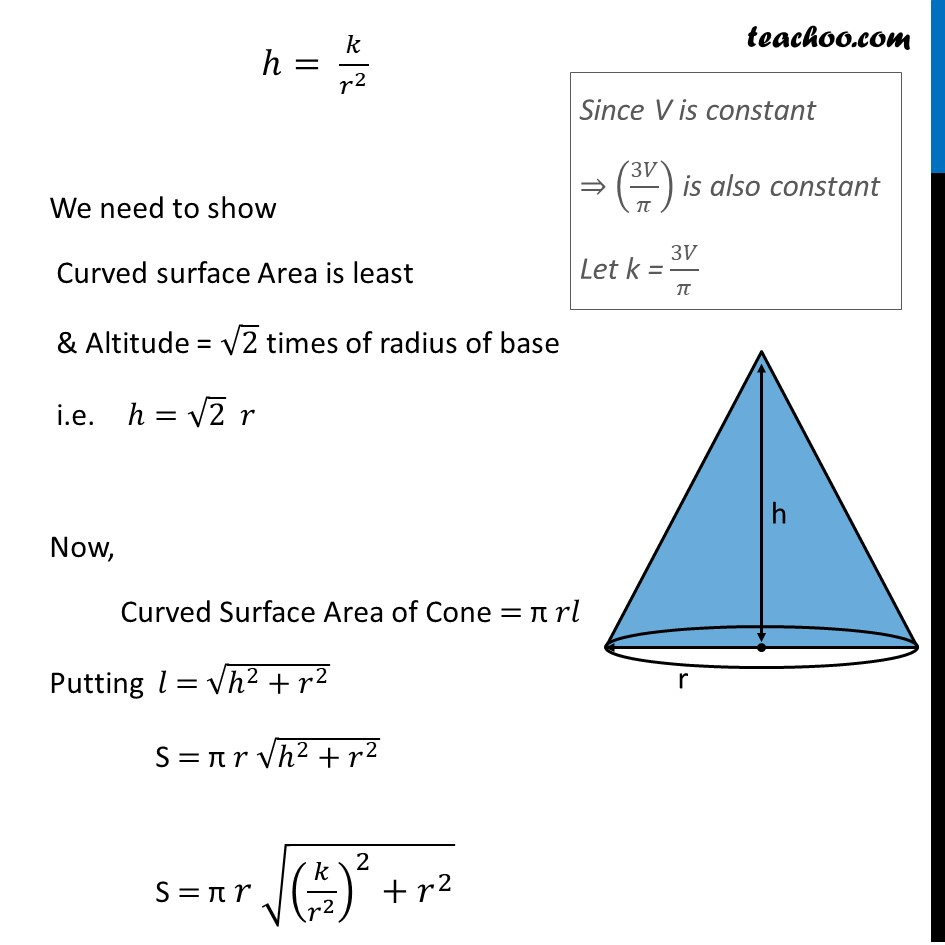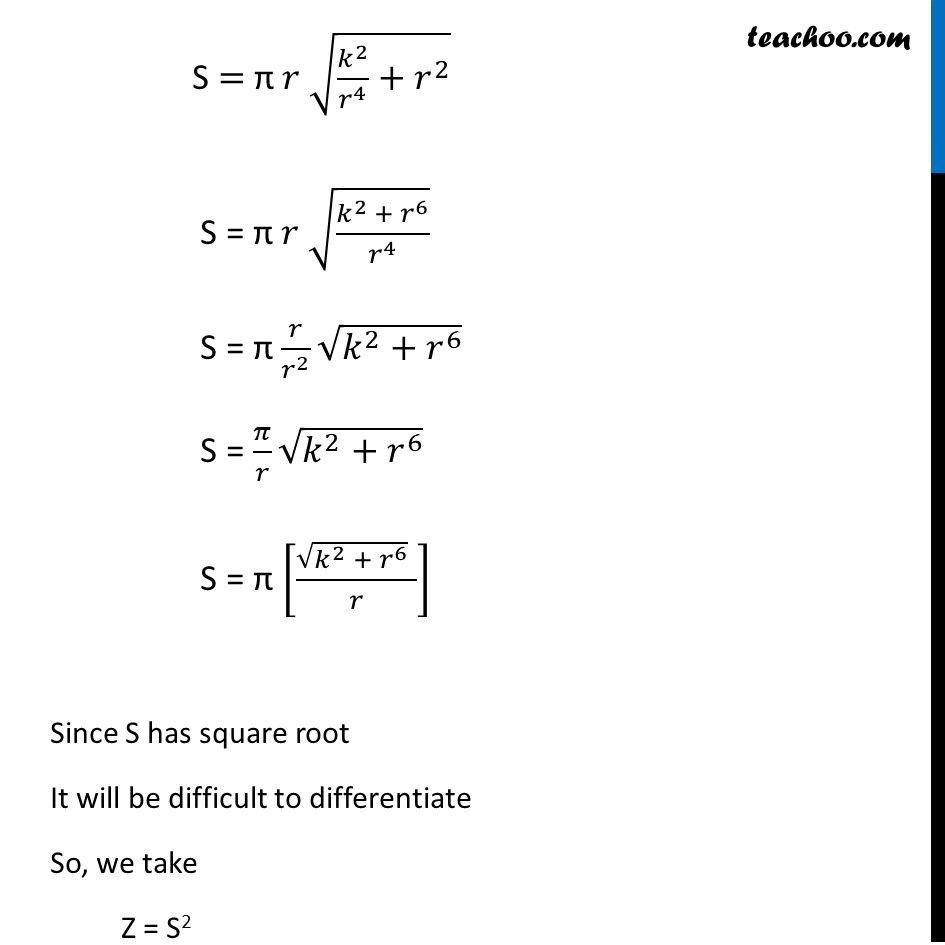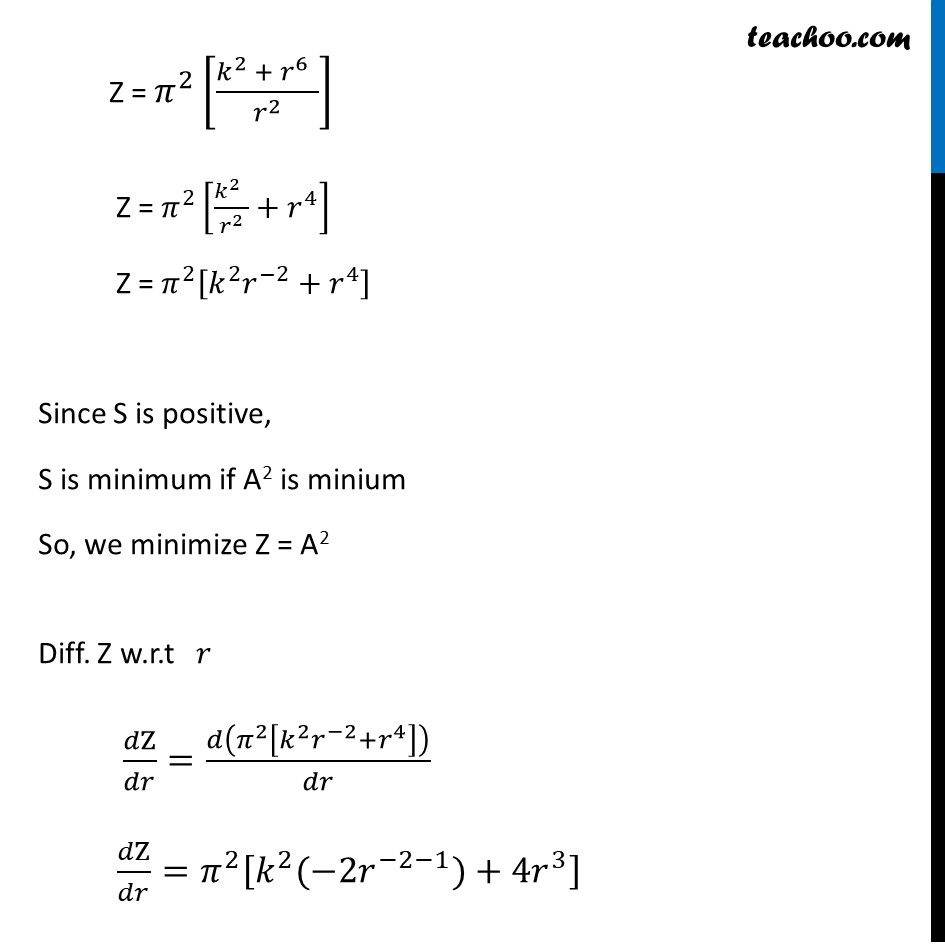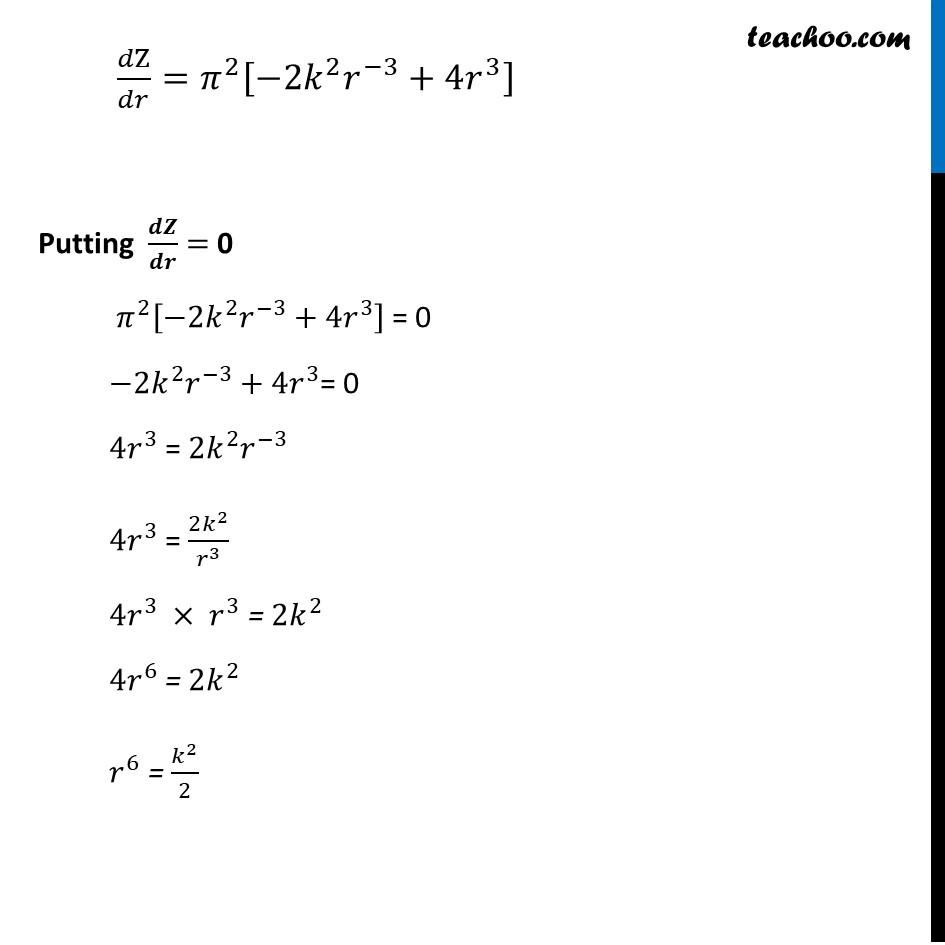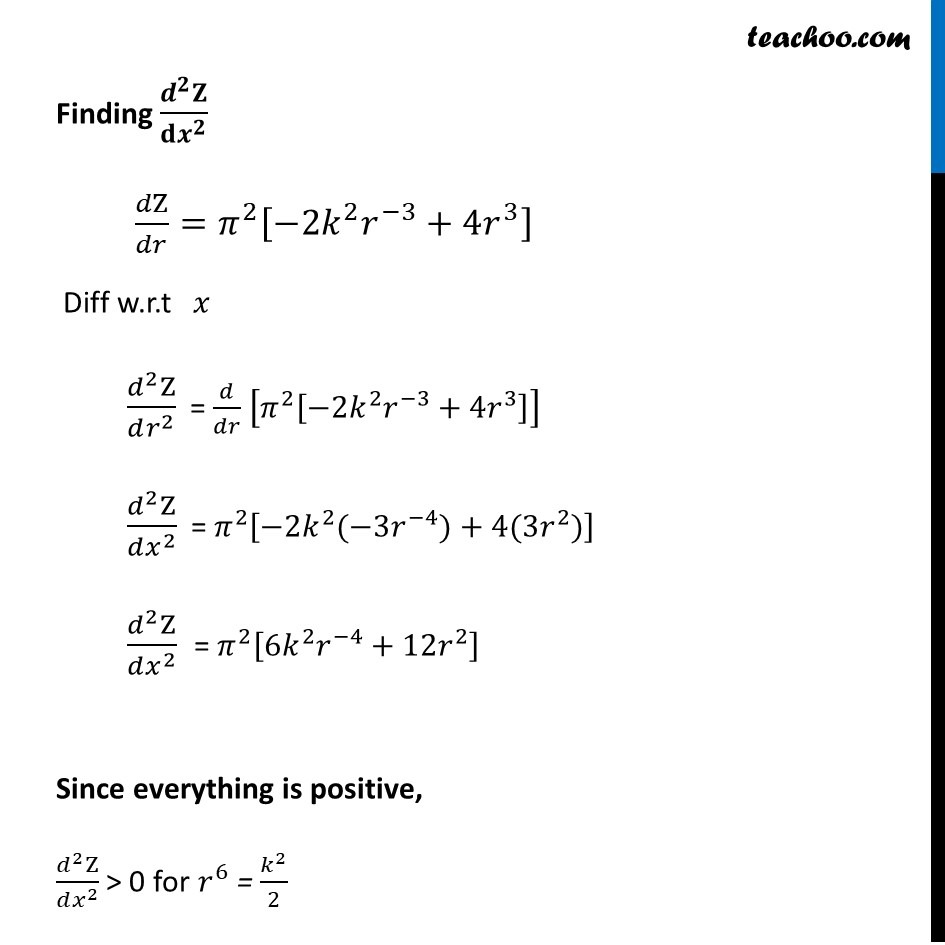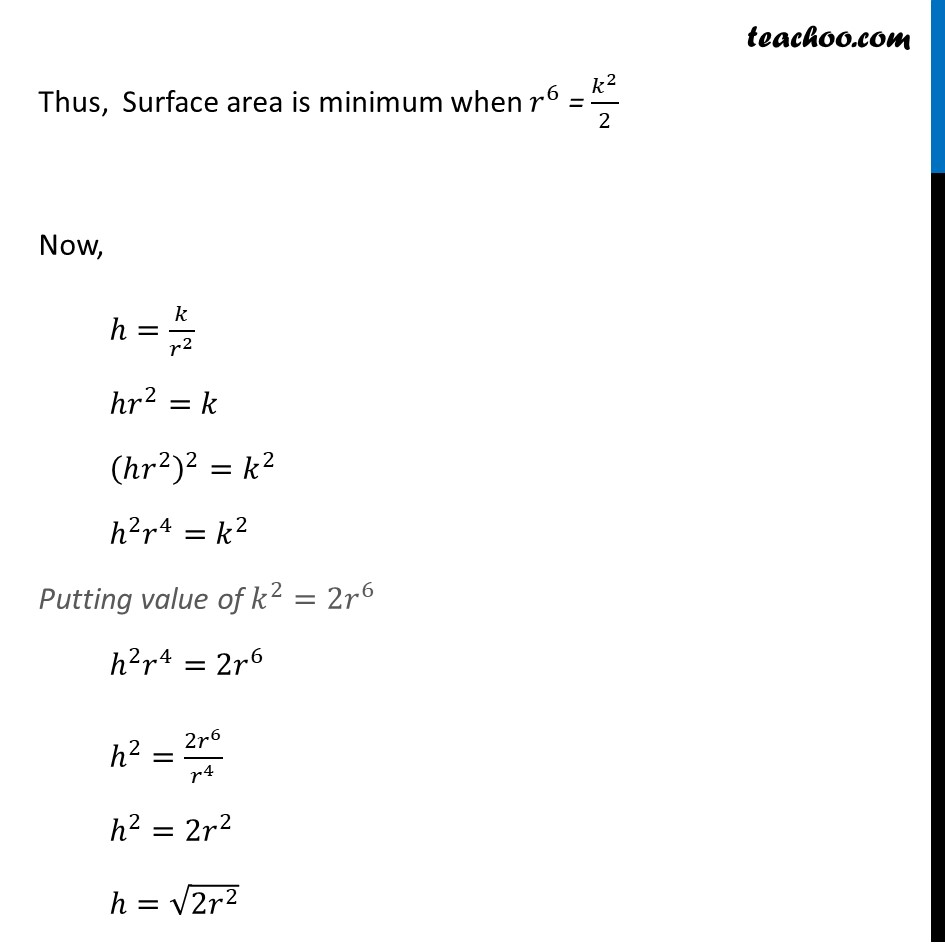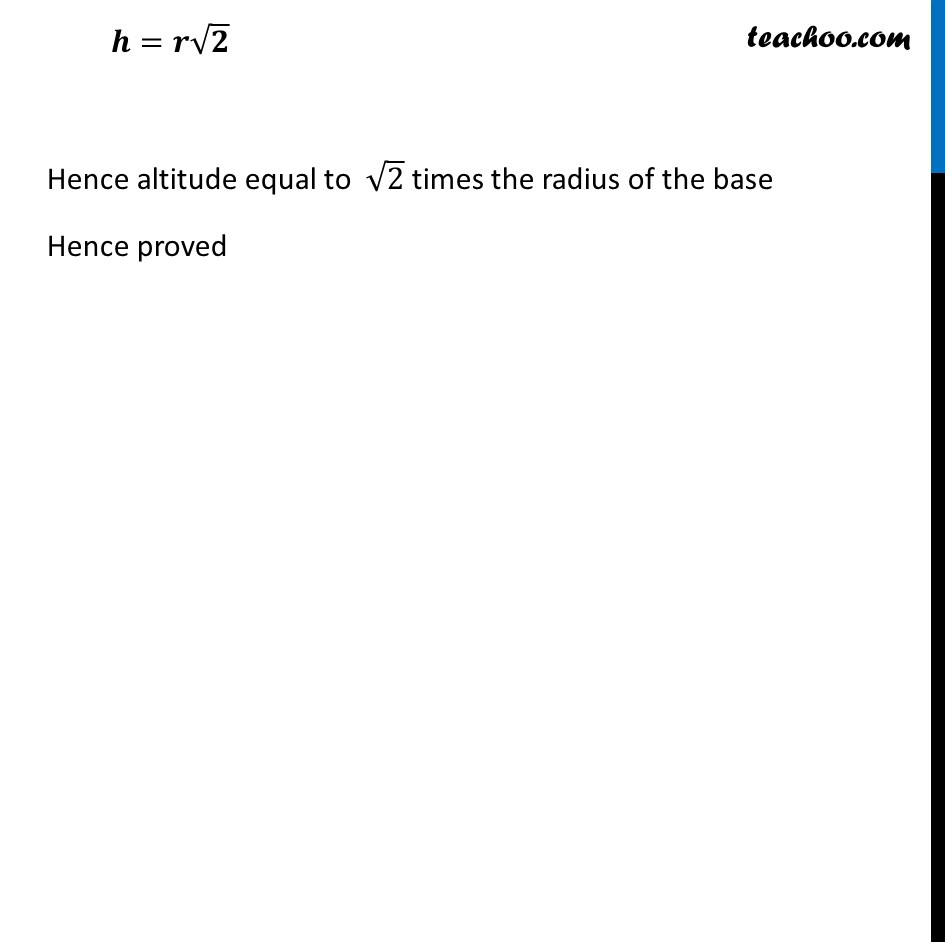Introducing your new favourite teacher - Teachoo Black, at only ₹83 per month

### Transcript

Ex 6.5, 24 Show that the right circular cone of least curved surface and given volume has an altitude equal to √2 time the radius of the baseLet r & h be the radius & height of a cone respectively And V & S be the volume & curved surface area of cone respectively Given volume of cone is constant Volume of cone = 1/3 𝜋(𝑟𝑎𝑑𝑖𝑢𝑠)^2 (ℎ𝑒𝑖𝑔ℎ𝑡) V = 1/3 𝜋(𝑟)^2 ℎ 3𝑉/𝜋=𝑟^2 ℎ h = (3𝑉/𝜋) 1/𝑟^2 ℎ= 𝑘/𝑟^2 We need to show Curved surface Area is least & Altitude = √2 times of radius of base i.e. ℎ=√2 𝑟 Now, Curved Surface Area of Cone = π 𝑟𝑙 Putting 𝑙 = √(ℎ^2+𝑟^2 ) S = π 𝑟 √(ℎ^2+𝑟^2 ) S = π 𝑟 √((𝑘/𝑟^2 )^2+𝑟^2 ) Since V is constant ⇒ (3𝑉/𝜋) is also constant Let k = 3𝑉/𝜋 S = π 𝑟 √(𝑘^2/𝑟^4 +𝑟^2 ) S = π 𝑟 √((𝑘^2 + 𝑟^6)/𝑟^4 ) S = π 𝑟/𝑟^2 √(𝑘^2+𝑟^6 ) S = 𝜋/𝑟 √(𝑘^2+𝑟^6 ) S = π [(√(𝑘^2 + 𝑟^6 ) " " )/𝑟] Since S has square root It will be difficult to differentiate So, we take Z = S2 Z = 𝜋^2 [(𝑘^2 + 𝑟^6 " " )/𝑟^2 ] Z = 𝜋^2 [(𝑘^2 " " )/𝑟^2 +𝑟^4 ] Z = 𝜋^2 [𝑘^2 𝑟^(−2)+𝑟^4 ] Since S is positive, S is minimum if A2 is minium So, we minimize Z = A2 Diff. Z w.r.t 𝑟 𝑑Z/𝑑𝑟=𝑑(𝜋^2 [𝑘^2 𝑟^(−2)+𝑟^4 ])/𝑑𝑟 𝑑Z/𝑑𝑟=𝜋^2 [𝑘^2 (−2𝑟^(−2−1))+4𝑟^3 ] 𝑑Z/𝑑𝑟=𝜋^2 [−2𝑘^2 𝑟^(−3)+4𝑟^3 ] Putting 𝒅𝒁/𝒅𝒓 = 0 𝜋^2 [−2𝑘^2 𝑟^(−3)+4𝑟^3 ] = 0 −2𝑘^2 𝑟^(−3)+4𝑟^3= 0 4𝑟^3 = 2𝑘^2 𝑟^(−3) 4𝑟^3 = (2𝑘^2)/𝑟^3 4𝑟^3 × 𝑟^3 = 2𝑘^2 4𝑟^6 = 2𝑘^2 𝑟^6 = 𝑘^2/2 Finding (𝒅^𝟐 𝐙)/(𝐝𝒙^𝟐 ) 𝑑Z/𝑑𝑟=𝜋^2 [−2𝑘^2 𝑟^(−3)+4𝑟^3 ] Diff w.r.t 𝑥 (𝑑^2 Z)/(𝑑𝑟^2 ) = 𝑑/𝑑𝑟 [𝜋^2 [−2𝑘^2 𝑟^(−3)+4𝑟^3 ]] (𝑑^2 Z)/(𝑑𝑥^2 ) = 𝜋^2 [−2𝑘^2 (−3𝑟^(−4))+4(3𝑟^2)] (𝑑^2 Z)/(𝑑𝑥^2 ) = 𝜋^2 [6𝑘^2 𝑟^(−4)+12𝑟^2 ] Since everything is positive, (𝑑^2 Z)/(𝑑𝑥^2 ) > 0 for 𝑟^6 = 𝑘^2/2 Thus, Surface area is minimum when 𝑟^6 = 𝑘^2/2 Now, ℎ=𝑘/𝑟^2 ℎ𝑟^2=𝑘 (ℎ𝑟^2 )^2=𝑘^2 ℎ^2 𝑟^4=𝑘^2 Putting value of 𝑘^2=2𝑟^6 ℎ^2 𝑟^4=2𝑟^6 ℎ^2=(2𝑟^6)/𝑟^4 ℎ^2=2𝑟^2 ℎ=√(2𝑟^2 ) 𝒉=𝒓√𝟐 Hence altitude equal to √2 times the radius of the base Hence proved